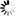DOI: 10.5176/2251-3388_2.1.40

Authors: M. Tlas

Abstract:
An efficient polynomial time algorithm for solving minimum cost flow problems has been proposed in this paper. This algorithm is basically based on successive divisions of capacities by multiples of two, and it solves the minimum cost flow problem as a sequence of O(n2)shortest path problems on residual networks with n nodes and runs in O(n2m r)time, where m is the number of arcs and r is the smallest integer greater than or equal to log B , and B is the largest arc capacity of the network. A numerical example is illustrated using the proposed algorithm.

Keywords: Minimum cost flow problem, Scaling algorithm, Polynomial time algorithm, Augmenting path method, Network flow

Price: \$0.00Updating...